Next: GF Numbering System. Up: Organization of the GF Previous: Organization of the GF

# Boundary Condition Types.

Five types of boundary conditions are defined at physical boundaries, and a zeroth'' type designates those cases with no physical boundaries. In the equations below the coordinate at the boundary is denoted ri and i indicates one of the boundaries. Type 1. Prescribed temperature (Dirichlet condition):

 T(ri, t ) = fi(ri, t )

Type 2. Prescribed heat flux (Neumann condition):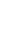k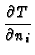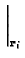= fi(ri, t )

Here ni is the outward-facing normal vector on the body surface.
Type 3. Convective boundary condition (sometimes called the Robin condition):k+ hiT(ri, t ) = fi(ri, t )

Here hi is the heat transfer coefficient and specified function fi is usually equal to hiT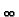where Tis a fluid temperature.
Type 4. Thin, high-conductivity film at the body surface:k= fi(ri, t ) - (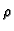cb)i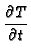Here product (cb)i are properties of the surface film (density, specific heat, and thickness), and the surface film must have a negligible temperature gradient across it (lumped'').
Type 5. Thin, high-conductivity film at the body surface, with the addition of convection heat losses from the surface:k+ hiT(ri, t ) = fi(ri, t ) - (cb)iType 0. No physical boundary. The number 0 (zero) is used where there is no physical boundary, which arises in several body shapes. For example, a semi-infinite body has boundary condition'' of type 0 at x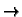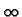. Another boundary'' of type 0 occurs at the center of a solid cylinder (or sphere), for which the coordinate has a limiting value (r = 0) but there is no physical boundary.Next: GF Numbering System. Up: Organization of the GF Previous: Organization of the GF
Kevin D. Cole
2003-07-21